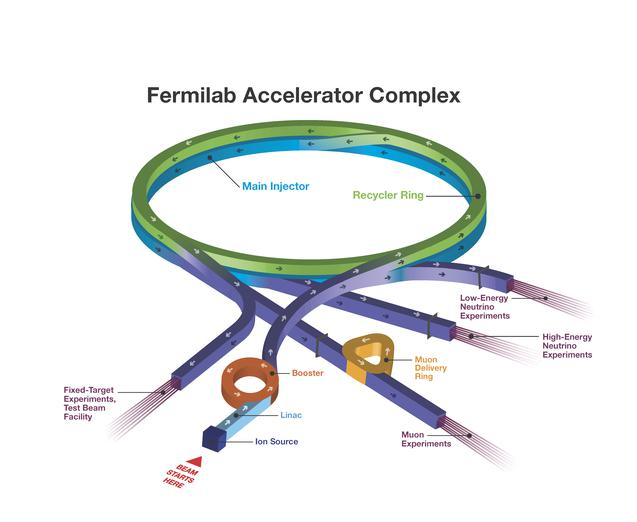# science based - Can an accelerator ring be used for energy ...

can the universe lose energy
habboin 22/09/2021 Universe 1826
Let me start by addressing the issue of magnets. The Large Hadron Collider, for example, requires 1232 dipole magnets generating magnetic fields of 8.3 tesla. I'm a little doubtful that rare earth mag...

Let me start by addressing the issue of magnets. The Large Hadron Collider, for example, requires 1232 dipole magnets generating magnetic fields of 8.3 tesla. I'm a little doubtful that rare earth magnets could achieve this properly; individually, they seem unable to achieve fields of more than ~1 tesla - nor are they capable of maintaining that field far enough from the magnets themselves. You could compensate for this by combining many together, but I'm not convinced that they would be adequate. I'm also not convinced that they could produce the field geometry you want, but I could be mistaken.

If we can't use rare earth magnets and have to resort to regular old superconducting magnets - well, that would kill the idea outright. These magnets have to be cooled to just 1.9 degrees above absolute zero. That costs money - and electricity. When the collider is being used, CERN requires 200 megawatts of power, i.e. roughly one-third of the power consumption of Geneva - and much of that power is not going into the particle beam.

Let's sidestep that particular difficulty. If you want to calculate the losses due to synchrotron radiation, the power emitted by a particle with charge $q$ and mass $m$ traveling a circular orbit with radius $r$ at an energy $E$, with a speed close to the speed of light, is approximately$$P\approx\frac{2kq^2(E/mc^2)^4c}{3r^2}=\frac{2kq^2E^4}{3m^4c^7r^2}$$For the LHC, setting $m$ and $q$ to be the mass of a proton, $r$ to be the LHC's radius (about 4.3 km) and $E$ to be 7 TeV, we get power a power loss per proton of $7.73\times10^{-12}$ Watts, which really isn't that bad. On the other hand, 7 TeV corresponds to about $10^{-6}$ Joules, so you're actually losing a significant amount of energy over the course of days to weeks (bearing in mind that $P$ will decrease as the particles lose energy). In short, we do have to consider energy losses - although you could mitigate this by increasing $r$ (yikes!).Could you recover this? Well, you'd need to take into account the spectrum of synchrotron radiation; higher energy particles have peak emission at higher photon energies, with an emission peak at a wavelength $\lambda_c$ that scales like$$\lambda_c\propto\frac{1}{E^2B}$$This could pose a challenge if you're trying to create something like a solar panel inside the beam tube - although I'd also be exceedingly worried about that solar-panel-esque device being hit by relativistic particles, as the beam isn't perfect! So perhaps a system based on directly absorbing photons would be problematic.

Finally, we have the issue of how much energy can be stored - and there are fundamental limits to how energetic individual particles in an accelerator's beams can be based on the properties of said accelerator. Higher energies require either stronger magnetic fields, larger accelerators or more particles per beam, which is presumably also limited by engineering constraints. I don't know what the ultimate limit is, but I'm sure there is one.

In short:

I'd want to see some evidence that the rare earth approach could work.If you use superconducting magnets, this whole thing is not going to be efficient.Just using the LHC as a model, synchrotron losses become important on the timescales of days or weeks.Maybe you could recoup that loss, but I don't see how. Normal photon-absorbing devices would risk being damaged.